"The most incomprehensible thing about the world is that
it is comprehensible."

- Einstein

AP Physics 1

Unit I - Mechanics - Study of the relationship between force and motionI. Kinematics - Mathematical methods of describing and predicting motion

Motion Plots

A. K. A.  Motion Diagrams(plots of motion information)

• Equations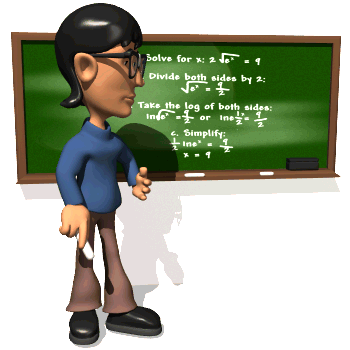II. How do we measure motion?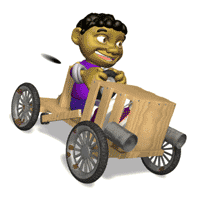Speed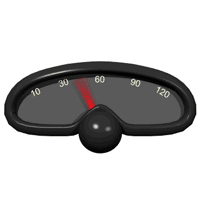AccelerationVelocity

(speed and direction)III. Measuring motion?Distance

(meter ≈ 1 yard)Time

(seconds)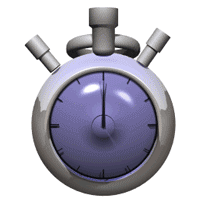DirectionA.  Speed - distance an object covers per unit time

1. Speed = distance / time

a) Units - m/s, km/hour, miles/hour

 A student on a merry-go-round  moves in a circular path with a radius of 3.5 m once every 8.9 secs. Student's average speed?       Average velocity?

= [2∏(3.5m)]/8.9 sec

= 2.5 m/s

Average velocity for a single trip around the merry-go-round?

0 m/s

 Ex 2) Cars A and B are 400. m apart. Car A travels 35. m/s east on a collision course with car B, which travels 15. m/s west.   How long will it take for the cars to collide?

When the cars collide, total d = 400. m

d = SpeedXtime

400.m = 35. m/s t + 15. m/s t

400 m = 50. m/s t

t = 8.0 s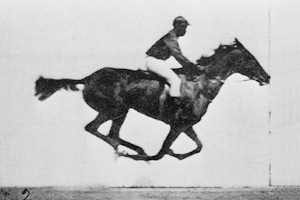Animation by User Waugsberg

Public Domain

 Ex 3) A car travels 250 km at an average speed of 95 km/h, stops for for lunch for 1.0 hour and then travels 250 km at a rate of 55 km/h. Car's average speed in m/s?

Speed = [total d]/[total time]

total d = 500 km

Total Time

Speed = d/t

Speedxtime = d

time = d/Speed

time 1 = 250 km/[95 km/h]

2.6 hour

time 2 - 1 hr

time 3 = 250 km/[55 km/h]

4.5 hour

Total t = 8.1 hours

Speed = [total d]/[total time]

Speed = 2(250 km)/8.1 hours

speed = 62 km/hr

[62 km/hr]x[1000m/km]x[1 hr/60 min]x[1 min/60 sec]

17 m/s

Ex 4) A cop riding 100 mph pursues a criminal travelling 85 mph. It takes the cop 15 minutes to catch up with the criminal. What distance, in miles, originally existed between the cop and the criminal when the pursuit originally began?

d = 15 mph x .25 hrs = 3.8 miles©Tony Mangiacapre., St. Mary's H.S., Manhasset, NY